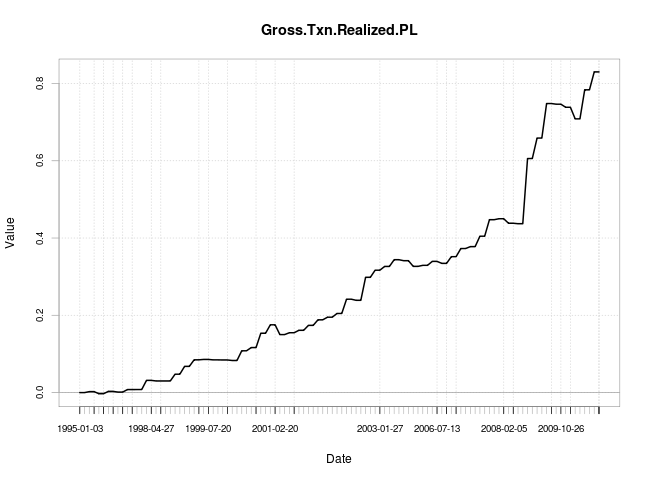Want to share your content on R-bloggers? click here if you have a blog, or here if you don't.

During last two sessions (December 23th and 27th), VIX index posted returns (close to close) above 6 %. My question is – what return can we expect next day after such event?

As you can see from the graph above, expected return is positive. During 1995-2010 were 53 such events and mean return was 1.02 % and median 0.6% and win rate 65%.
What can be the explanation for such consistency in returns? It is known, that volatility is mean reverting process and the value of VIX index tends to return to its mean.Worth to note, that despite VIX index spike, S&P 500 index was very very still during the last days.

?View Code RSPLUS

 ```  require('xts') require('quantmod') require('blotter') require('PerformanceAnalytics') require('FinancialInstrument') Sys.setenv(TZ="GMT")   #data part getSymbols(c('SPY','^VIX'),from='1995-01-01',index.class=c("POSIXt","POSIXct")) dividends<-getDividends('SPY',from='1995-01-01',index.class=c("POSIXt","POSIXct"))   temp<-cbind(dividends,SPY) temp[,1][is.na(temp[,1])]<-0   SPY<-cbind(temp[,2],temp[,3],temp[,4],temp[,1]+temp[,5]) colnames(SPY)<-c('Open','High','Low','Close') spy.delta<-Delt(Cl(SPY))   vix.delta<-Delt(Cl(VIX))   signal<-ifelse(vix.delta>0.06& lag(vix.delta>0.06,1),1,0) png('2highdays.png',width=650) chart.CumReturns(lag(signal,1)*spy.delta,main='when VIX >6% during two days') dev.off()   #blotter code symbols<-c('SPY') SPY<-Cl(SPY) initDate=time(get(symbols)) initEq=50000 rm(list=ls(envir=.blotter),envir=.blotter) ltportfolio='2high' ltaccount='2high' initPortf(ltportfolio,symbols, initDate=initDate) initAcct(ltaccount,portfolios=c(ltportfolio), initDate=initDate,initEq=initEq) currency("USD") stock(symbols,currency="USD",multiplier=1)   signal[is.na(signal)]<-0     for(i in 2:length(signal)) { currentDate= time(signal)[i] equity = initEq #getEndEq(ltaccount, currentDate) position = getPosQty(ltportfolio, Symbol=symbols, Date=currentDate) print(position) print(currentDate) if(position==0) { #open a new position if signal is >0 if(signal[i]>0 ) { print('open position') closePrice<-as.double(Cl(SPY[currentDate])) print(closePrice) unitSize = as.numeric(trunc((equity/closePrice))) print(unitSize) commssions=-unitSize*closePrice*0.0003 addTxn(ltportfolio, Symbol=symbols, TxnDate=currentDate, TxnPrice=closePrice, TxnQty = unitSize , TxnFees=commssions, verbose=T)   }   } else { #position is open. If signal is 0 - close it. if(position>0 &signal[i] ==0) { position = getPosQty(ltportfolio, Symbol=symbols, Date=currentDate) closePrice<-as.double((Cl(SPY[currentDate])))#as.double(get(symbols)[i+100]) commssions=-position*closePrice*0.0003 addTxn(ltportfolio, Symbol=symbols, TxnDate=currentDate, TxnPrice=closePrice, TxnQty = -position , TxnFees=commssions, verbose=T)   }   } updatePortf(ltportfolio, Dates = currentDate) updateAcct(ltaccount, Dates = currentDate) updateEndEq(ltaccount, Dates = currentDate) } rez1<-(getPortfolio(ltaccount)) png('2highdays_2.png',width=650) chart.CumReturns(rez1\$symbols\$SPY\$txn[,7]/initEq) dev.off()```